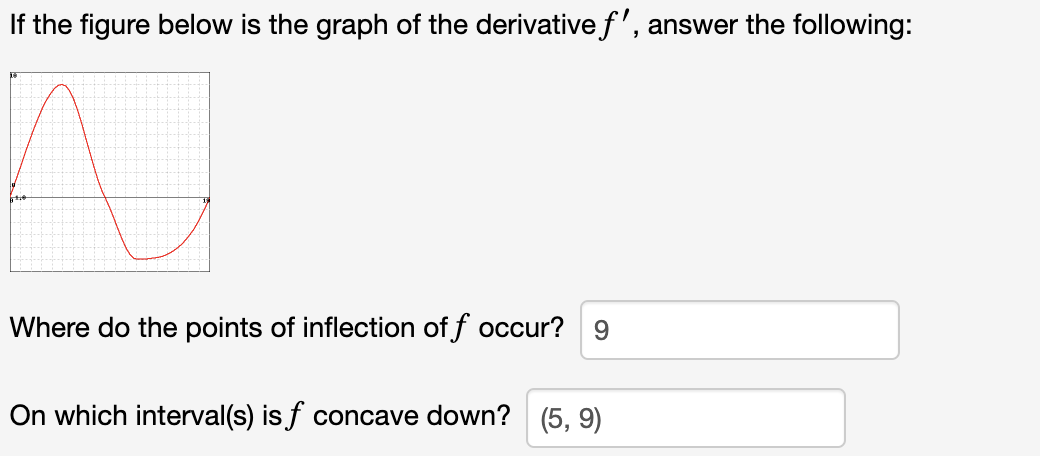# If the figure below is the graph of the derivativef', answer the following:Where do the points of inflection off occur?On which interval(s) is f concave down?(5, 9)

Question
50 viewshelp_outlineImage TranscriptioncloseIf the figure below is the graph of the derivativef', answer the following: Where do the points of inflection off occur? On which interval(s) is f concave down? (5, 9) fullscreen
check_circle

Step 1

The point of inflection are the points where the function changes its behaviour that is sign.

The point of the inflection is given by the points where f’’(x) = 0.

And at these points the slope of the derivative of function f’’ is 0.

From the graph it is clearly that the tangents line at the top and bottom of the graph that means par...

### Want to see the full answer?

See Solution

#### Want to see this answer and more?

Solutions are written by subject experts who are available 24/7. Questions are typically answered within 1 hour.*

See Solution
*Response times may vary by subject and question.
Tagged in

### Other